# Mean State

Period Mean (original grids) [W/m2]
Model Period Mean (intersection) [W/m2]
Model Period Mean (complement) [W/m2]
Benchmark Period Mean (intersection) [W/m2]
Benchmark Period Mean (complement) [W/m2]
Bias [W/m2]
RMSE [W/m2]
Phase Shift [months]
Bias Score 
RMSE Score 
Seasonal Cycle Score 
Spatial Distribution Score 
Interannual Variability Score 
Overall Score 
Benchmark [-] 457.
CLM4 [-] 458. 458. 0.00 456. 465. 3.03 16.8 1.30 0.42 0.37 0.81 0.81 0.63 0.57
CLM4.5 [-] 462. 462. 0.00 456. 465. 6.98 18.2 1.33 0.38 0.37 0.81 0.83 0.63 0.56
CLM5 [-] 464. 464. 0.00 456. 465. 8.32 17.6 1.41 0.42 0.38 0.80 0.88 0.62 0.58
Period Mean (original grids) [W/m2]
Model Period Mean (intersection) [W/m2]
Model Period Mean (complement) [W/m2]
Benchmark Period Mean (intersection) [W/m2]
Benchmark Period Mean (complement) [W/m2]
Bias [W/m2]
RMSE [W/m2]
Phase Shift [months]
Bias Score 
RMSE Score 
Seasonal Cycle Score 
Spatial Distribution Score 
Interannual Variability Score 
Overall Score 
Benchmark [-] 466.
CLM4 [-] 480. 480. 0.00 467. 458. 13.8 22.7 0.636 0.51 0.48 0.95 0.98 0.67 0.68
CLM4.5 [-] 485. 485. 0.00 467. 458. 18.7 25.1 0.646 0.43 0.49 0.94 1.0 0.66 0.67
CLM5 [-] 487. 487. 0.00 467. 458. 20.4 26.5 0.743 0.41 0.48 0.93 1.0 0.64 0.66
Period Mean (original grids) [W/m2]
Model Period Mean (intersection) [W/m2]
Model Period Mean (complement) [W/m2]
Benchmark Period Mean (intersection) [W/m2]
Benchmark Period Mean (complement) [W/m2]
Bias [W/m2]
RMSE [W/m2]
Phase Shift [months]
Bias Score 
RMSE Score 
Seasonal Cycle Score 
Spatial Distribution Score 
Interannual Variability Score 
Overall Score 
Benchmark [-] 443.
CLM4 [-] 446. 446. 0.00 443. 446. 4.22 18.5 1.00 0.56 0.49 0.87 0.97 0.66 0.67
CLM4.5 [-] 452. 452. 0.00 443. 446. 9.23 19.1 1.06 0.54 0.49 0.87 0.98 0.67 0.67
CLM5 [-] 450. 450. 0.00 443. 446. 7.78 19.3 1.24 0.56 0.47 0.85 0.99 0.63 0.66
Period Mean (original grids) [W/m2]
Model Period Mean (intersection) [W/m2]
Model Period Mean (complement) [W/m2]
Benchmark Period Mean (intersection) [W/m2]
Benchmark Period Mean (complement) [W/m2]
Bias [W/m2]
RMSE [W/m2]
Phase Shift [months]
Bias Score 
RMSE Score 
Seasonal Cycle Score 
Spatial Distribution Score 
Interannual Variability Score 
Overall Score 
Benchmark [-] 279.
CLM4 [-] 260. 260. 0.00 278. 296. -15.8 23.6 0.135 0.78 0.77 0.99 0.99 0.74 0.84
CLM4.5 [-] 262. 262. 0.00 278. 296. -14.5 21.5 0.132 0.80 0.79 0.99 0.99 0.72 0.84
CLM5 [-] 268. 268. 0.00 278. 296. -8.32 17.5 0.132 0.86 0.80 0.99 1.0 0.71 0.86
Period Mean (original grids) [W/m2]
Model Period Mean (intersection) [W/m2]
Model Period Mean (complement) [W/m2]
Benchmark Period Mean (intersection) [W/m2]
Benchmark Period Mean (complement) [W/m2]
Bias [W/m2]
RMSE [W/m2]
Phase Shift [months]
Bias Score 
RMSE Score 
Seasonal Cycle Score 
Spatial Distribution Score 
Interannual Variability Score 
Overall Score 
Benchmark [-] 457.
CLM4 [-] 450. 450. 0.00 454. 467. -1.91 13.4 1.90 0.37 0.37 0.70 0.90 0.55 0.54
CLM4.5 [-] 452. 452. 0.00 454. 467. -0.886 13.6 1.88 0.35 0.38 0.71 0.89 0.55 0.54
CLM5 [-] 457. 457. 0.00 454. 467. 3.95 14.6 1.88 0.30 0.37 0.71 0.92 0.54 0.54
Period Mean (original grids) [W/m2]
Model Period Mean (intersection) [W/m2]
Model Period Mean (complement) [W/m2]
Benchmark Period Mean (intersection) [W/m2]
Benchmark Period Mean (complement) [W/m2]
Bias [W/m2]
RMSE [W/m2]
Phase Shift [months]
Bias Score 
RMSE Score 
Seasonal Cycle Score 
Spatial Distribution Score 
Interannual Variability Score 
Overall Score 
Benchmark [-] 312.
CLM4 [-] 304. 304. 0.00 311. 334. -7.50 12.3 0.0422 0.89 0.87 1.0 0.99 0.86 0.91
CLM4.5 [-] 304. 304. 0.00 311. 334. -7.01 12.3 0.0406 0.90 0.87 1.0 1.0 0.86 0.91
CLM5 [-] 311. 310. 0.00 311. 334. -0.679 9.44 0.0515 0.94 0.89 1.0 0.99 0.86 0.93
Period Mean (original grids) [W/m2]
Model Period Mean (intersection) [W/m2]
Model Period Mean (complement) [W/m2]
Benchmark Period Mean (intersection) [W/m2]
Benchmark Period Mean (complement) [W/m2]
Bias [W/m2]
RMSE [W/m2]
Phase Shift [months]
Bias Score 
RMSE Score 
Seasonal Cycle Score 
Spatial Distribution Score 
Interannual Variability Score 
Overall Score 
Benchmark [-] 364.
CLM4 [-] 357. 357. 0.00 363. 399. -4.21 14.3 0.128 0.85 0.82 0.99 1.0 0.82 0.88
CLM4.5 [-] 358. 357. 0.00 363. 399. -3.48 14.3 0.116 0.85 0.82 0.99 1.0 0.82 0.88
CLM5 [-] 363. 362. 0.00 363. 399. 0.922 14.0 0.225 0.86 0.82 0.98 1.0 0.80 0.88
Period Mean (original grids) [W/m2]
Model Period Mean (intersection) [W/m2]
Model Period Mean (complement) [W/m2]
Benchmark Period Mean (intersection) [W/m2]
Benchmark Period Mean (complement) [W/m2]
Bias [W/m2]
RMSE [W/m2]
Phase Shift [months]
Bias Score 
RMSE Score 
Seasonal Cycle Score 
Spatial Distribution Score 
Interannual Variability Score 
Overall Score 
Benchmark [-] 448.
CLM4 [-] 453. 453. 0.00 446. 467. 8.82 19.0 0.579 0.57 0.57 0.95 1.0 0.71 0.73
CLM4.5 [-] 457. 457. 0.00 446. 467. 12.5 20.9 0.590 0.52 0.57 0.94 1.0 0.71 0.72
CLM5 [-] 459. 459. 0.00 446. 467. 14.4 21.7 0.742 0.51 0.56 0.92 1.0 0.67 0.71
Period Mean (original grids) [W/m2]
Model Period Mean (intersection) [W/m2]
Model Period Mean (complement) [W/m2]
Benchmark Period Mean (intersection) [W/m2]
Benchmark Period Mean (complement) [W/m2]
Bias [W/m2]
RMSE [W/m2]
Phase Shift [months]
Bias Score 
RMSE Score 
Seasonal Cycle Score 
Spatial Distribution Score 
Interannual Variability Score 
Overall Score 
Benchmark [-] 281.
CLM4 [-] 268. 268. 0.00 281. 288. -12.4 21.7 0.0955 0.82 0.74 0.99 0.98 0.80 0.85
CLM4.5 [-] 268. 268. 0.00 281. 288. -12.4 19.4 0.136 0.82 0.78 0.99 0.98 0.77 0.85
CLM5 [-] 275. 276. 0.00 281. 288. -4.81 13.7 0.101 0.91 0.81 0.99 0.99 0.78 0.88
Period Mean (original grids) [W/m2]
Model Period Mean (intersection) [W/m2]
Model Period Mean (complement) [W/m2]
Benchmark Period Mean (intersection) [W/m2]
Benchmark Period Mean (complement) [W/m2]
Bias [W/m2]
RMSE [W/m2]
Phase Shift [months]
Bias Score 
RMSE Score 
Seasonal Cycle Score 
Spatial Distribution Score 
Interannual Variability Score 
Overall Score 
Benchmark [-] 441.
CLM4 [-] 447. 447. 0.00 443. 426. 1.56 13.2 0.363 0.79 0.70 0.97 0.97 0.83 0.83
CLM4.5 [-] 447. 447. 0.00 443. 426. 1.85 13.5 0.356 0.78 0.70 0.97 0.97 0.83 0.82
CLM5 [-] 449. 449. 0.00 443. 426. 3.69 13.5 0.384 0.78 0.70 0.97 0.98 0.80 0.82
Period Mean (original grids) [W/m2]
Model Period Mean (intersection) [W/m2]
Model Period Mean (complement) [W/m2]
Benchmark Period Mean (intersection) [W/m2]
Benchmark Period Mean (complement) [W/m2]
Bias [W/m2]
RMSE [W/m2]
Phase Shift [months]
Bias Score 
RMSE Score 
Seasonal Cycle Score 
Spatial Distribution Score 
Interannual Variability Score 
Overall Score 
Benchmark [-] 368.
CLM4 [-] 358. 358. 0.00 366. 389. -4.71 13.8 0.275 0.81 0.75 0.98 0.99 0.82 0.85
CLM4.5 [-] 358. 358. 0.00 366. 389. -5.26 13.3 0.253 0.81 0.76 0.98 0.99 0.82 0.85
CLM5 [-] 362. 363. 0.00 366. 389. -0.870 12.0 0.447 0.85 0.77 0.97 1.0 0.80 0.86
Period Mean (original grids) [W/m2]
Model Period Mean (intersection) [W/m2]
Model Period Mean (complement) [W/m2]
Benchmark Period Mean (intersection) [W/m2]
Benchmark Period Mean (complement) [W/m2]
Bias [W/m2]
RMSE [W/m2]
Phase Shift [months]
Bias Score 
RMSE Score 
Seasonal Cycle Score 
Spatial Distribution Score 
Interannual Variability Score 
Overall Score 
Benchmark [-] 399.
CLM4 [-] 373. 373. 0.00 376. 409. -1.28 16.7 0.501 0.70 0.67 0.94 1.0 0.71 0.78
CLM4.5 [-] 375. 375. 0.00 376. 409. -0.0436 17.0 0.506 0.69 0.67 0.94 1.0 0.71 0.78
CLM5 [-] 378. 378. 0.00 376. 409. 3.26 16.3 0.561 0.71 0.67 0.94 1.0 0.69 0.78
Period Mean (original grids) [W/m2]
Model Period Mean (intersection) [W/m2]
Model Period Mean (complement) [W/m2]
Benchmark Period Mean (intersection) [W/m2]
Benchmark Period Mean (complement) [W/m2]
Bias [W/m2]
RMSE [W/m2]
Phase Shift [months]
Bias Score 
RMSE Score 
Seasonal Cycle Score 
Spatial Distribution Score 
Interannual Variability Score 
Overall Score 
Benchmark [-] 450.
CLM4 [-] 455. 455. 0.00 450. 446. 5.46 16.3 0.342 0.81 0.76 0.97 0.99 0.73 0.84
CLM4.5 [-] 455. 455. 0.00 450. 446. 5.39 16.4 0.340 0.81 0.76 0.97 0.99 0.72 0.84
CLM5 [-] 457. 457. 0.00 450. 446. 6.78 16.8 0.377 0.81 0.76 0.97 0.99 0.71 0.83
Period Mean (original grids) [W/m2]
Model Period Mean (intersection) [W/m2]
Model Period Mean (complement) [W/m2]
Benchmark Period Mean (intersection) [W/m2]
Benchmark Period Mean (complement) [W/m2]
Bias [W/m2]
RMSE [W/m2]
Phase Shift [months]
Bias Score 
RMSE Score 
Seasonal Cycle Score 
Spatial Distribution Score 
Interannual Variability Score 
Overall Score 
Benchmark [-] 281.
CLM4 [-] 271. 271. 0.00 281. 293. -10.3 18.2 0.200 0.84 0.78 0.98 0.98 0.71 0.85
CLM4.5 [-] 272. 272. 0.00 281. 293. -9.92 17.8 0.194 0.84 0.79 0.98 0.98 0.70 0.85
CLM5 [-] 278. 278. 0.00 281. 293. -3.57 15.7 0.202 0.90 0.79 0.98 0.99 0.71 0.86
Period Mean (original grids) [W/m2]
Model Period Mean (intersection) [W/m2]
Model Period Mean (complement) [W/m2]
Benchmark Period Mean (intersection) [W/m2]
Benchmark Period Mean (complement) [W/m2]
Bias [W/m2]
RMSE [W/m2]
Phase Shift [months]
Bias Score 
RMSE Score 
Seasonal Cycle Score 
Spatial Distribution Score 
Interannual Variability Score 
Overall Score 
Benchmark [-] 442.
CLM4 [-] 446. 445. 0.00 439. 459. 11.0 19.8 0.839 0.50 0.53 0.89 0.99 0.73 0.69
CLM4.5 [-] 444. 444. 0.00 439. 459. 9.15 18.6 0.833 0.53 0.54 0.89 1.0 0.72 0.70
CLM5 [-] 446. 446. 0.00 439. 459. 11.3 19.0 0.815 0.51 0.55 0.90 1.0 0.69 0.70
Period Mean (original grids) [W/m2]
Model Period Mean (intersection) [W/m2]
Model Period Mean (complement) [W/m2]
Benchmark Period Mean (intersection) [W/m2]
Benchmark Period Mean (complement) [W/m2]
Bias [W/m2]
RMSE [W/m2]
Phase Shift [months]
Bias Score 
RMSE Score 
Seasonal Cycle Score 
Spatial Distribution Score 
Interannual Variability Score 
Overall Score 
Benchmark [-] 386.
CLM4 [-] 381. 381. 0.00 384. 429. -0.942 9.59 0.124 0.89 0.85 0.99 0.99 0.89 0.91
CLM4.5 [-] 382. 382. 0.00 384. 429. 0.154 10.0 0.112 0.89 0.84 0.99 0.99 0.89 0.91
CLM5 [-] 388. 388. 0.00 384. 429. 6.07 11.6 0.309 0.86 0.85 0.98 0.99 0.87 0.90
Period Mean (original grids) [W/m2]
Model Period Mean (intersection) [W/m2]
Model Period Mean (complement) [W/m2]
Benchmark Period Mean (intersection) [W/m2]
Benchmark Period Mean (complement) [W/m2]
Bias [W/m2]
RMSE [W/m2]
Phase Shift [months]
Bias Score 
RMSE Score 
Seasonal Cycle Score 
Spatial Distribution Score 
Interannual Variability Score 
Overall Score 
Benchmark [-] 324.
CLM4 [-] 315. 316. 0.00 323. 347. -6.68 11.5 0.0546 0.87 0.84 1.0 1.0 0.79 0.89
CLM4.5 [-] 316. 316. 0.00 323. 347. -5.85 11.4 0.0600 0.88 0.84 1.0 1.0 0.79 0.89
CLM5 [-] 323. 323. 0.00 323. 347. 0.502 10.9 0.135 0.91 0.84 0.99 0.99 0.78 0.89
Period Mean (original grids) [W/m2]
Model Period Mean (intersection) [W/m2]
Model Period Mean (complement) [W/m2]
Benchmark Period Mean (intersection) [W/m2]
Benchmark Period Mean (complement) [W/m2]
Bias [W/m2]
RMSE [W/m2]
Phase Shift [months]
Bias Score 
RMSE Score 
Seasonal Cycle Score 
Spatial Distribution Score 
Interannual Variability Score 
Overall Score 
Benchmark [-] 377.
CLM4 [-] 373. 374. 0.00 377. 378. -2.73 14.9 0.0869 0.82 0.81 0.99 0.96 0.82 0.87
CLM4.5 [-] 374. 375. 0.00 377. 378. -2.11 15.1 0.0899 0.82 0.81 0.99 0.97 0.84 0.87
CLM5 [-] 378. 379. 0.00 377. 378. 2.18 14.4 0.110 0.84 0.81 0.99 0.98 0.80 0.87
Period Mean (original grids) [W/m2]
Model Period Mean (intersection) [W/m2]
Model Period Mean (complement) [W/m2]
Benchmark Period Mean (intersection) [W/m2]
Benchmark Period Mean (complement) [W/m2]
Bias [W/m2]
RMSE [W/m2]
Phase Shift [months]
Bias Score 
RMSE Score 
Seasonal Cycle Score 
Spatial Distribution Score 
Interannual Variability Score 
Overall Score 
Benchmark [-] 449.
CLM4 [-] 453. 453. 0.00 449. 463. 4.25 13.2 1.08 0.41 0.40 0.86 0.89 0.68 0.61
CLM4.5 [-] 455. 455. 0.00 449. 463. 6.56 14.3 1.07 0.36 0.40 0.86 0.89 0.69 0.60
CLM5 [-] 456. 456. 0.00 449. 463. 7.54 14.0 1.07 0.35 0.41 0.86 0.95 0.67 0.61
Period Mean (original grids) [W/m2]
Model Period Mean (intersection) [W/m2]
Model Period Mean (complement) [W/m2]
Benchmark Period Mean (intersection) [W/m2]
Benchmark Period Mean (complement) [W/m2]
Bias [W/m2]
RMSE [W/m2]
Phase Shift [months]
Bias Score 
RMSE Score 
Seasonal Cycle Score 
Spatial Distribution Score 
Interannual Variability Score 
Overall Score 
Benchmark [-] 443.
CLM4 [-] 445. 444. 0.00 443. 434. 0.796 15.0 0.770 0.67 0.56 0.89 0.97 0.73 0.73
CLM4.5 [-] 447. 447. 0.00 443. 434. 3.31 15.4 0.843 0.65 0.56 0.88 0.96 0.74 0.72
CLM5 [-] 449. 449. 0.00 443. 434. 4.97 13.9 0.865 0.70 0.57 0.88 0.99 0.69 0.73
Period Mean (original grids) [W/m2]
Model Period Mean (intersection) [W/m2]
Model Period Mean (complement) [W/m2]
Benchmark Period Mean (intersection) [W/m2]
Benchmark Period Mean (complement) [W/m2]
Bias [W/m2]
RMSE [W/m2]
Phase Shift [months]
Bias Score 
RMSE Score 
Seasonal Cycle Score 
Spatial Distribution Score 
Interannual Variability Score 
Overall Score 
Benchmark [-] 383.
CLM4 [-] 380. 378. 0.00 381. 396. -1.53 21.1 0.906 0.61 0.56 0.89 1.0 0.66 0.71
CLM4.5 [-] 382. 381. 0.00 381. 396. 0.459 21.0 0.916 0.62 0.56 0.89 1.0 0.67 0.71
CLM5 [-] 383. 383. 0.00 381. 396. 2.21 20.5 0.925 0.63 0.57 0.88 1.0 0.65 0.72

# Temporally integrated period mean

BENCHMARK MEAN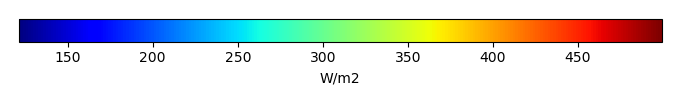MODEL MEANBIAS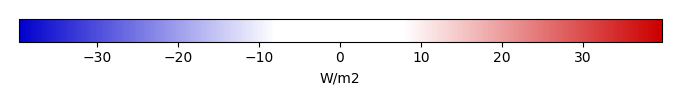BIAS SCORERMSE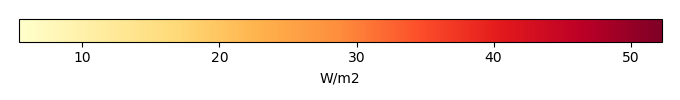RMSE SCOREBENCHMARK INTERANNUAL VARIABILITY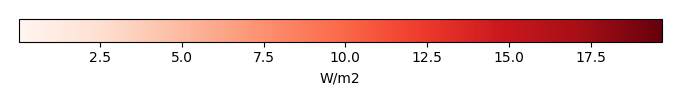MODEL INTERANNUAL VARIABILITYINTERANNUAL VARIABILITY SCOREBENCHMARK MAX MONTHMODEL MAX MONTHDIFFERENCE IN MAX MONTH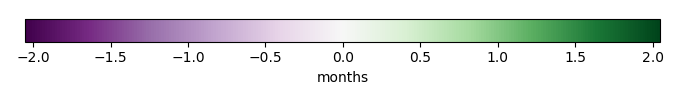SEASONAL CYCLE SCORESPATIAL TAYLOR DIAGRAMMODEL COLORS# Spatially integrated regional mean

MODEL COLORSREGIONAL MEANANNUAL CYCLEMONTHLY ANOMALYANNUAL CYCLE# All Models

BenchmarkCLM4CLM4.5CLM5# Data Information

creation_date: Thu May 8 23:13:11 PDT 2014

source_file: This product is generated from monthly 1 degree CERES EBAF Radiation observations